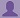SHARE
HELP

To start with, Sr2Jr’s first step is to reduce the expenses related to education. To achieve this goal Sr2Jr organized the textbook’s question and answers. Sr2Jr is community based and need your support to fill the question and answers. The question and answers posted will be available free of cost to all.# Calculus Early Transcendentals

Authors:
James Stewart
Exercise:
Review
Chapter:
Functions And Models
Edition:
7
ISBN:
9780538497909
Question:
5
 Previous Next

###Question

What is a mathematical model?

###Answer

A mathematical model is a mathematical description (often by means of a function or an equation) of a real-world phenomenon such as

-the size of a population

-the demand for a product

-the speed of a falling object

-the concentration of a product in a chemical reaction

-the life expectancy of a person at birth, or

-the cost of emission reductions.

The purpose of constructing the model is to understand the phenomenon and to make predictions about future behavior. The model helps in finding the solution to the problems for which it is constructed.

### Discussions

Post the discussion to improve the above solution.